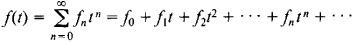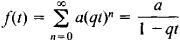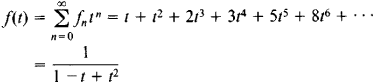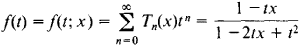# Generating Function

Also found in: Acronyms, Wikipedia.

## generating function

[′jen·ə‚rād·iŋ ‚fəŋk·shən]
(mathematics)
A function g (x, y) corresponding to a family of orthogonal polynomials ƒ0(x), ƒ1(x),…, where a Taylor series expansion of g (x, y) in powers of y will have the polynomial ƒn (x) as the coefficient for the term y n .
A function, g (y), corresponding to a sequence a0, a1, ⋯) where g (y) = a0+ a1 y + a2 y 2+ …. Also known as ordinary generating function.
McGraw-Hill Dictionary of Scientific & Technical Terms, 6E, Copyright © 2003 by The McGraw-Hill Companies, Inc.
The following article is from The Great Soviet Encyclopedia (1979). It might be outdated or ideologically biased.

## Generating Function

A generating function of the sequence f0, f1,..., fn is the functionassuming that this power series converges for at least one nonzero value of t.

The sequence f0, f1,..., fn,… can be a sequence of numbers or of functions. In the latter case, the generating function depends not only on t but also on the arguments of the functions fn. For example, if fn = aqn, where a and q are constants, the generating function isIf the fn are Fibonacci numbers—that is, if f0 = 0, f1 = 1, fn+2 = fn+1 + fn—we haveIf fn = Tn (x) are Chebyshev polynomials—that is, if T0(x) = 1

and Tn (x) = cos (n arc cos x)—thenKnowledge of the generating function of a sequence often makes it easier to study the properties of the sequence. Generating functions are used in probability theory, in the theory of functions, and in the theory of invariants in algebra. Methods involving generating functions were first applied by P. Laplace to solve certain problems in probability theory.

### REFERENCES

Feller, W. Vvedenie v teoriiu veroiatnostei i eeprilozheniia, 2nd ed., vols. 1–2. Moscow, 1967. (Translated from English.)
Natanson, I. P. Konstruktivnaia teoriia funktsii. Moscow-Leningrad, 1949.
References in periodicals archive ?
According to the 2017 NDAA, the purpose of institutional capacity-building is "to enhance the capacity of such foreign country to organize, administer, employ, manage, maintain, sustain, or oversee the national security forces of such foreign country." Institutional capacity-building can also be likened to defense institution-building, which is the doctrinal term used in JP 3-20 to describe SFA at the executive and generating functions of a foreign security force.
to define a extension of the generalized Apostol type polynomials in x, parameters c, a, [mu], v, order [alpha], and level m through the following generating function:
In this section, we derive the joint moment generating function of the underlying log-asset price and counterparty' logasset value.
This viewpoint comes from interpreting the function as a generating function enumerating objects in some combinatorial class.
[g.sup.(i).sub.n] (0) i--fold convolution of {[g.sub.n]} with itself and [g.sup.(0).sub.n] = [[delta].sub.n,0] [g.sup.(i).sub.n] = [n-1.summation over (k=1)] [g.sup.(i-1).sub.n-k] [g.sub.k] where [[delta].sub.m,n] is the Kroncker--Delta function X(z) Probability generating function of X.
say, where P(x) = P(x; [alpha]) is the residual function which is the sum of the residues of the generating function, [phi](s) to be defined below, with weights and we are to express the error term [DELTA](x; [delta]) in terms of special functions and where the prime on the summation sign means that the term corresponding to ([n.sub.1] + [[alpha].sub.1]) ...
Moreover, inverse hazard, the information generating, moment generating functions were introduced and studied.
The generating function is [mathematical expression not reproducible], where N is main term and P is a small perturbation.
For convenience, if we change the running number n = l' - m', this generating function can also be modified as
Next, define the generating function [G.sub.j](s,t) = [[summation].sub.i[greater than or equal to]0] [p.sub.ij](t), which is finite if [absolute value of s] < 1.
Let [M.sub.i,k](x) be the exponential generating function of the numbers [m.sub.i,k](n).
In particular, the generating function of F is g (x/(1 - x)).

Site: Follow: Share:
Open / Close